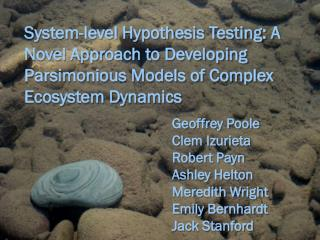# System-level Hypothesis Testing: A Novel Approach to Developing Parsimonious Models of Complex Ecosystem Dynamics - PowerPoint PPT PresentationDownload PresentationSystem-level Hypothesis Testing: A Novel Approach to Developing Parsimonious Models of Complex Ecosystem Dynamics

System-level Hypothesis Testing: A Novel Approach to Developing Parsimonious Models of Complex Ecosystem DynamicsDownload Presentation## System-level Hypothesis Testing: A Novel Approach to Developing Parsimonious Models of Complex Ecosystem Dynamics

- - - - - - - - - - - - - - - - - - - - - - - - - - - E N D - - - - - - - - - - - - - - - - - - - - - - - - - - -
##### Presentation Transcript

1. System-level Hypothesis Testing: A Novel Approach to Developing Parsimonious Models of Complex Ecosystem Dynamics Geoffrey Poole Clem Izurieta Robert Payn Ashley Helton Meredith Wright Emily Bernhardt Jack Stanford

2. SE techniques yield: Model parsimony Code reliability, extensibility, reuse, longevity Grid-based high-performance computing Virtual laboratories An Imperative Scheller et al. 2009

3. Virtual laboratory; model parsimony • Requires: rapid creation, maintenance, management, and execution of multiple competing model variants • Code reliability, extensibility, and reuse • Requires: encapsulation and modularization of code • High performance computing • Requires: A simple means of developing multi-threaded code The NEED

4. What IS A Model? Umax Uptake k [Solute] Biomass [Solute] Time

5. NEO Rethinking models... Simulation run Values Code r = 0.034 Input/Output/ Initialization u = 6.2 Ks = 1.73 Model Execution control W = 600 U = 17.3 Calculations R = 6.5 X = 13.4 S =1800.0 [S] = 3.00

6. CREATING A NEO SIMULATION Solution Biomass Uptake Respiration E2 E1 C1 C2 NEO INPUT FILE: Matrix Table

7. Ks W µ r E2 E1 C1 C2 NEO INPUT FILE: Parameter file

8. Solution Biomass Uptake Respiration eq4 Ks S W eq5 µ r [S] E2 E1 C1 C2 NEO INPUT FILE: Holon Type Table Model code hierarchy eq2 4 NEO INPUT FILE: Cell Type Behavior Table R eq3 5 eq1 X U 3 NEO INPUT FILE: Edge Type Behavior Table 1 2

9. Solution Biomass Uptake Respiration eq4 Ks S W eq5 µ r [S] E2 E1 C1 C2 Biomass eq2 R eq3 eq1 X U [Solute] Time

10. Solution Biomass eq4 Ks S W eq5 µ r [S] E2 E1 “MyEdge” Uptake Respiration “FromCell” “ToCell” C1 C2 Model code hierarchy Pseudo code public class Carbon extends Dynam { private StateVal Ks, u, conc, X; public double initialize() { Ks = myEdge.getStateVal(“Ks”); u = myEdge.getStateVal(“u”); conc = fromCell.getStateVal(“[S]”); X = toCell.getStateVal(“X”); return 0.0; } public double calculate() { return u.v * (Ks.v / (Ks.v + conc.v)) * X.v; } } eq2 4 R eq3 5 eq1 X U 3 1 2

11. Multiple Interactive currencies Microbial uptake and respiration “HorzGroundwaterFlux” S Q Ks S W µ r [S] Resp Uptake Biomass S Q Water flux and solute transport Ks S W µ R R R r [S] X X X U U U S Q Ks S W µ r [S] S Q

12. Code Reuse and recombination SurfaceWater Surface Water Flux SWFlux Biomass Biomass H20 DOC SWFlux Horizontal GW Flux Areal Uptake OC GWSW Exchange CO2 Horizontal GWFlux Respiration Horizontal GWFlux Areal Uptake SurfaceWater H2O DOC Biomass OC M-M Uptake M-M Uptake CO2 Respiration GroundWater Respiration Advective Solute Flux CO2 GWSW Exchange

13. Parallel Processing Step 2: Use parallel processing to expedite run-time of individual model executions. Speeds applications of highly detailed or complex models. Step 1: Perform multiple independent model executions (with different parameters) simultaneously across multiple processing threads. Speeds applications such as sensitivity analyses. NEO model spawner Model execution Model executions Processing threads Processing threads Model output Model outputs

14. As a modeling framework, NEO allows: • Specification of model parameters, structure, and algorithms at run time • NEO facilitates: • Rapid model development • Simple management of code complexity • Addition and removal of model dynamics • Applications on HPC platforms • Maintenance, management and execution of multiple model variants The bottom line…

15. Hypothesis testing requires making predictions • At a whole-system level, a model is required • Models variants are competing hypotheses • Complexity can be added/removed • Alternative behaviors can be substituted • Empirical data are used to reject models • Last model standing (if any) is supported System Level Hypothesis testing

16. Sources of error in model assessment • Empirical data • Model parameters • Model structure • Bayesian approaches presume that model structure is fixed. • NEO opens the possibility of Bayesian assessment to determine the probability of alternative model structures. SYNERGY with Bayesian approaches?

17. http://www.montana.edu/FLL

18. A) Model B) Illustrative code public class Carbon extends Dynam { private StateValconc, water; public double initialize() { conc = fromCell.getStateVal(“CarbonConc”); water = myEdge.getStateVal(“Water”); return 0.0; } public double calculate() { return conc * water; } } Model Packages 4 public class Carbon extends Dynam { private StateVal k, u_max, conc, biomass; public double initialize() { k = myholon.getStateVal(“K”); u_max = myEdge.getStateVal(“u_max”); conc = fromCell.getStateVal(“CarbonConc”); biomass = toCell.getStateVal(“Carbon”); return 0.0; } public double calculate() { return u_max * (k / (k + conc)) * biomass; } } Holons 5 1 Behaviors 3 public class Carbon extends Dynam { private StateVal r, biomass; public double initialize() { r = myEdge.getStateVal(“r”); biomass = fromCell.getStateVal(“Carbon”); return 0.0; } public double calculate() { return -r * biomass; } } 2 1 Deviant 2

19. Ecosystems aSMulti-Currency Flux Nets Model of solute transport/processing (currencies are water, heat, carbon, nitrogen, oxygen) within a linked hydro-biological system (network) Helton et al. Accepted

20. PHYSICAL SYSTEMS as Flux networks Surface and ground water (currency) flux across and through the Nyack Floodplain, Middle Fork Flathead River (network) Poole et al. 2006

21. Biotic communities as Flux networks Baxter et al. 2005 Food web (network) representing carbon (currency) flux